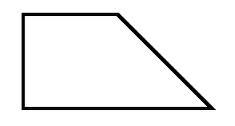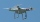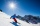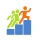# Trapezoid MO

The rectangular trapezoid ABCD with right angle at point B, |AC| = 12, |CD| = 8, diagonals are perpendicular to each other.

Calculate the perimeter and area of ​​the trapezoid.

Correct result:

p =  33.31
A =  69.25

#### Solution:

$|AC| = 12 \ \\ |CD| = 8 \ \\ \ \\ \sin \Theta = \dfrac{|BC|}{|AC|} \ \\ \cos \Theta = \dfrac{|BC|}{|BD|} \ \\ \ \\ \cos^2 \Theta + \dfrac{ |CD|}{|AC|}\cos \Theta - 1 =0 \ \\ x^2 + \dfrac{ |CD|}{|AC|}x - 1 =0 \ \\ \ \\ x^2 +0.667x -1 =0 \ \\ \ \\ a=1; b=0.667; c=-1 \ \\ D = b^2 - 4ac = 0.667^2 - 4\cdot 1 \cdot (-1) = 4.4444444444 \ \\ D>0 \ \\ \ \\ x_{1,2} = \dfrac{ -b \pm \sqrt{ D } }{ 2a } = \dfrac{ -0.67 \pm \sqrt{ 4.44 } }{ 2 } \ \\ x_{1,2} = -0.33333333 \pm 1.05409255339 \ \\ x_{1} = 0.720759220056 \ \\ x_{2} = -1.38742588672 \ \\ \ \\ \text{ Factored form of the equation: } \ \\ (x -0.720759220056) (x +1.38742588672) = 0 \ \\ \ \\ \ \\ \Theta = 43^\circ 52'58" \ \\ \ \\ |BC| = |AC| \sin \Theta = 8.31822608044 \ \\ |AB| = |AC| \cos \Theta = 8.64911064067 \ \\ |AD| = \sqrt{ |BC|^2 + (|AB|-|CD|)^2} = 8.34351423258 \ \\ \ \\ o = |AB|+|BC|+|CD| + |AD| = 33.31$
$A=\frac{\left(\mathrm{\mid }AB\mathrm{\mid }+\mathrm{\mid }CD\mathrm{\mid }\right)\cdot \mathrm{\mid }BC\mathrm{\mid }}{2}=69.25$Our examples were largely sent or created by pupils and students themselves. Therefore, we would be pleased if you could send us any errors you found, spelling mistakes, or rephasing the example. Thank you!

Please write to us with your comment on the math problem or ask something. Thank you for helping each other - students, teachers, parents, and problem authors.

Showing 1 comment:Math student
How do you find the answerTips to related online calculators
Looking for help with calculating roots of a quadratic equation?
Need help calculate sum, simplify or multiply fractions? Try our fraction calculator.
Do you have a linear equation or system of equations and looking for its solution? Or do you have quadratic equation?
Pythagorean theorem is the base for the right triangle calculator.

#### You need to know the following knowledge to solve this word math problem:

We encourage you to watch this tutorial video on this math problem:

## Next similar math problems:

• The basesThe bases of the isosceles trapezoid ABCD have lengths of 10 cm and 6 cm. Its arms form an angle α = 50˚ with a longer base. Calculate the circumference and content of the ABCD trapezoid.
• Traffic signThere is a traffic sign for climbing on the road with an angle of 7%. Calculate at what angle the road rises (falls).
• Octagonal pyramidFind the volume of a regular octagonal pyramid with height v = 100 and the angle of the side edge with the plane of the base is α = 60°.
• Tetrahedral pyramid 8Let’s all side edges of the tetrahedral pyramid ABCDV be equally long and its base let’s be a rectangle. Determine its volume if you know the deviations A=40° B=70° of the planes of adjacent sidewalls and the plane of the base and the height h=16 of the p
• A droneA flying drone aimed the area for an architect. He took off perpendicularly from point C to point D. He was at a height of 300 m above the plane of ABC. The drone from point D pointed at a BDC angle of 43°. Calculate the distance between points C and B in
• Power line poleFrom point A, the power line pole is seen at an angle of 18 degrees. From point B to which we get when going from point A 30m away from the column at an angle of 10 degrees. Find the height of the power pole.
• Black diamond runTaleah is skiing down a black diamond run. She begins skiing at the top of a ski trail whose elevation is about 8625 feet. The ski run ends toward the base of the mountain at 3800 feet. The horizontal distance between these two points is about 4775 feet.
• An observerAn observer standing west of the tower sees its top at an altitude angle of 45 degrees. After moving 50 meters to the south, he sees its top at an altitude angle of 30 degrees. How tall is the tower?
• The towerThe observer sees the base of the tower 96 meters high at a depth of 30 degrees and 10 minutes and the top of the tower at a depth of 20 degrees and 50 minutes. How high is the observer above the horizontal plane on which the tower stands?
• FighterA military fighter flies at an altitude of 10 km. From the ground position, it was aimed at an altitude angle of 23° and 12 seconds later at an altitude angle of 27°. Calculate the speed of the fighter in km/h.
• TV towerCalculate the height of the television tower if an observer standing 430 m from the base of the tower sees the peak at an altitude angle of 23°?The ladder touch on a wall at a height of 7.5 m. The angle of the inclination of the ladder is 76°. How far is the lower end of the ladder from the wall?From the observation tower at a height of 105 m above sea level, the ship is aimed at a depth angle of 1° 49´. How far is the ship from the base of the tower?The rescue helicopter is above the landing site at a height of 180m. The site of the rescue operation can be seen from here at a depth angle of 52° 40 '. How far will the helicopter land from the rescue site?Find the height between the two floors if you know that the number of steps between the two floors is 18, the gradient is 30º and the length of the step is 28.6 cm. Report the result in centimeters to the nearest centimeter.Calculate the volume and area of the cone whose height is 10 cm and the axial section of the cone has an angle of 30 degrees between height and the cone side.Calculate the volume (V) and the surface (S) of a regular quadrilateral prism whose height is 28.6 cm and the deviation of the body diagonal from the base plane is 50°.